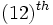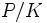# Maximal among abelian characteristic subgroups may be multiple and isomorphic

## Statement

It is possible to have a group of prime power order$P$ with two distinct subgroups$H, K \le P$, such that both$H$ and$K$ are Maximal among abelian characteristic subgroups (?), and$H \cong K$.

## Facts used

1. Classification of abelian subgroups of maximum order in unipotent upper-triangular matrix groups

## Related facts

The example described here also shows many other things:

## Proof

### Example involving the upper triangular matrices

Suppose$p$ is any prime, and let$G := U(5,p)$ be the group of upper-triangular unipotent$5 \times 5$ matrices over the field of$p$ elements. Let$P$ be the subgroup of$G$ comprising those matrices where the$(12)^{th}$ entry is zero. Then,$P$ is a group of order$p^9$.

By fact (1), we have that$G$ has two subgroups that are Abelian of maximum order: the rectangle groups of dimensions$2 \times 3$ and$3 \times 2$ respectively. Call these subgroups$H$ and$K$ respectively. Then, observe that:

• Both$H$ and$K$ are also Abelian subgroups of maximum order in$P$. Moreover, they are the only Abelian subgroups of maximum order in$P$ since they are the only Abelian subgroups of maximum order in$G$.
•$H$ and$K$ are isomorphic -- in fact, they are conjugate subgroups inside the bigger group$GL(5,p)$. This conjugation restricts to an automorphism of$G$, but not of$P$.
• Both$H$ and$K$ are normal in$G$, and hence in$P$. The quotient$P/H$ is isomorphic to the unipotent subgroup of 3-by-3 matrices while the quotient$P/K$ is isomorphic to the elementary Abelian group of order$p^3$. Hence,$H$ and$K$ are not automorphic subgroups in$P$.
• Thus,$H$ and$K$ are the only Abelian subgroups of their order, and they are not automorphic in$P$. Hence, they are both characteristic subgroups. Since they are Abelian of maximum order, they are both maximal among Abelian characteristic subgroups.

We have thus established all the required conditions.